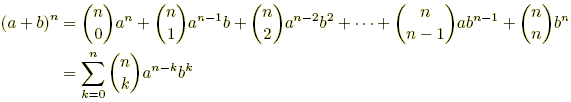index: click on a letter A B C D E F G H I J K L M N O P Q R S T U V W X Y Z A to Z index index: subject areas numbers & symbols sets, logic, proofs geometry algebra trigonometry advanced algebra & pre-calculus calculus advanced topics probability & statistics real world applications multimedia entrieswww.mathwords.com about mathwords website feedback

Binomial Theorem

A method for distributing powers of binomials as shown below.

 Formula:Example: (a + b)4 = a4 + 4a3b + 6a2b2 + 4ab3 + b4 The coefficients are from the fourth row of Pascal's Triangle as shown below.